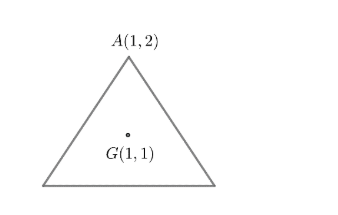Courses
Courses for Kids
Free study material
Free LIVE classes
More# The centroid and a vertex of an equilateral triangle are $\left( 1,1 \right)$and $\left( 1,2 \right)$ respectively. Another vertex of the triangle can beA) $\left( \dfrac{2-\sqrt{3}}{2},\dfrac{1}{2} \right)$ B) $\left( \dfrac{2+3\sqrt{3}}{2},\dfrac{1}{2} \right)$C) $\left( \dfrac{2+\sqrt{3}}{2},\dfrac{1}{2} \right)$D) None of these

Last updated date: 25th Mar 2023
Total views: 308.4k
Views today: 6.85kVerified
308.4k+ views
Hint: Centroid is the intersection point of medians. Orthocentre is the intersection point of altitudes. Circumcentre is the intersection point of perpendicular bisectors of sides of a triangle.

In the case of an equilateral triangle, median, altitude, and perpendicular bisector are the same. Hence, centroid, circumcentre, and orthocentre coincide.
In the case of an equilateral triangle, we know that centroid, orthocentre, and circumcentre coincide.
We also know that the circumcentre is equidistant from the vertices.
So , in the case of an equilateral triangle, the centroid is equidistant from the vertices.
Now, the given centroid is $G\left( 1,1 \right)$and one vertex is $A\left( 1,2 \right)$.We, know the distance between two points $\left( {{x}_{1}},{{y}_{1}} \right)$ and $\left( {{x}_{2}},{{y}_{2}} \right)$ is given as;
$\sqrt{{{\left( {{x}_{1}}-{{x}_{2}} \right)}^{2}}+{{\left( {{y}_{1}}-{{y}_{2}} \right)}^{2}}}$
So, $AG=\sqrt{{{\left( 1-1 \right)}^{2}}+{{\left( 2-1 \right)}^{2}}}=1$
So, $A{{G}^{2}}=1$
Now, since $G$ is the centroid as well as the circumcentre, the other two vertices should lie on a circle with $G\left( 1,1 \right)$as the centre and $AG=1$ as the radius.
Now, we know that the equation of a circle with center at $\left( a,b \right)$ and radius$=r$ is given as
${{\left( x-a \right)}^{2}}+{{\left( y-b \right)}^{2}}={{r}^{2}}$
So, the equation of circumcircle becomes
${{\left( x-1 \right)}^{2}}+{{\left( y-1 \right)}^{2}}=1..........\left( i \right)$
So, the other two vertices should lie on this circle .
Now , we will check the options .
Option (a) :$\left( \dfrac{2-\sqrt{3}}{2},\dfrac{1}{2} \right)$
We will substitute $\left( \dfrac{2-\sqrt{3}}{2},\dfrac{1}{2} \right)$ in equation $\left( i \right)$.
On substituting $\left( \dfrac{2-\sqrt{3}}{2},\dfrac{1}{2} \right)$in equation $\left( i \right)$, we get;
\begin{align} & {{\left( \dfrac{2-\sqrt{3}}{2}-1 \right)}^{2}}+{{\left( \dfrac{1}{2}-1 \right)}^{2}}=1 \\ & \Rightarrow {{\left( \dfrac{-\sqrt{3}}{2} \right)}^{2}}+{{\left( \dfrac{-1}{2} \right)}^{2}}=1 \\ & \Rightarrow \dfrac{3}{4}+\dfrac{1}{4}=1 \\ & \Rightarrow \dfrac{4}{4}=1 \\ & \Rightarrow 1=1 \\ & LHS=RHS \\ \end{align}
So ,$\left( \dfrac{2-\sqrt{3}}{2},\dfrac{1}{2} \right)$ satisfies the equation and hence , can be one of the vertices .
Option (b): $\left( \dfrac{2+3\sqrt{3}}{2},\dfrac{1}{2} \right)$
Now , we will substitute $\left( \dfrac{2+3\sqrt{3}}{2},\dfrac{1}{2} \right)$in equation $\left( i \right)$.
On substituting $\left( \dfrac{2+3\sqrt{3}}{2},\dfrac{1}{2} \right)$in equation $\left( i \right)$ we get,
\begin{align} & {{\left( \dfrac{2+3\sqrt{3}}{2}-1 \right)}^{2}}+{{\left( \dfrac{1}{2}-1 \right)}^{2}}=1 \\ & \Rightarrow {{\left( \dfrac{3\sqrt{3}}{2} \right)}^{2}}+{{\left( \dfrac{-1}{2} \right)}^{2}}=1 \\ & \Rightarrow \dfrac{27}{4}+\dfrac{1}{4}=1 \\ & \Rightarrow \dfrac{28}{4}=1 \\ & \Rightarrow 7=1 \\ \end{align}
Which is false i.e. $LHS\ne RHS$
So , $\left( \dfrac{2+3\sqrt{3}}{2},\dfrac{1}{2} \right)$ does not satisfy the equation and hence cannot be the vertex of the triangle.
Option (c): $\left( \dfrac{2+\sqrt{3}}{2},\dfrac{1}{2} \right)$
Now , we will substitute $\left( \dfrac{2+\sqrt{3}}{2},\dfrac{1}{2} \right)$in equation$\left( i \right)$.
On substituting $\left( \dfrac{2+\sqrt{3}}{2},\dfrac{1}{2} \right)$in equation $\left( i \right)$, we get
\begin{align} & {{\left( \dfrac{2+\sqrt{3}}{2}-1 \right)}^{2}}+{{\left( \dfrac{1}{2}-1 \right)}^{2}}=1 \\ & \Rightarrow {{\left( \dfrac{\sqrt{3}}{2} \right)}^{2}}+{{\left( \dfrac{-1}{2} \right)}^{2}}=1 \\ & \Rightarrow \dfrac{3}{4}+\dfrac{1}{4}=1 \\ & \Rightarrow 1=1 \\ \end{align}
Which is true i.e. $LHS=RHS$
Hence , $\left( \dfrac{2+\sqrt{3}}{2},\dfrac{1}{2} \right)$can be a vertex of the triangle.
Therefore, $\left( \dfrac{2+\sqrt{3}}{2},\dfrac{1}{2} \right)$ or $\left( \dfrac{2-\sqrt{3}}{2},\dfrac{1}{2} \right)$ can be a vertex of the triangle.
Option (a) $\left( \dfrac{2-\sqrt{3}}{2},\dfrac{1}{2} \right)$(c) $\left( \dfrac{2+\sqrt{3}}{2},\dfrac{1}{2} \right)$ are correct.

Note: The distance between two points $({{x}_{1}},{{y}_{1}})$and $({{x}_{2}},{{y}_{2}})$ is given as $d=\sqrt{{{({{x}_{1}}-{{x}_{2}})}^{2}}+{{({{y}_{1}}-{{y}_{2}})}^{2}}}$ and not $d=\sqrt{{{({{x}_{1}}+{{x}_{2}})}^{2}}+{{({{y}_{1}}+{{y}_{2}})}^{2}}}$. It is a very common mistake made by students.